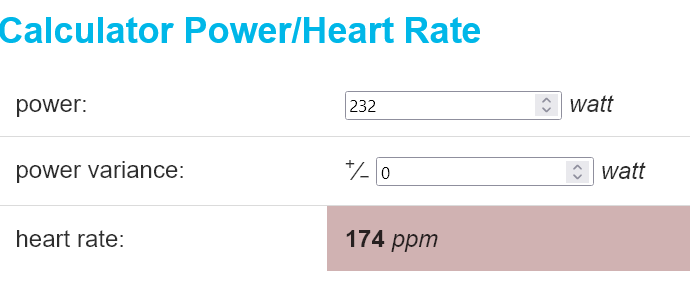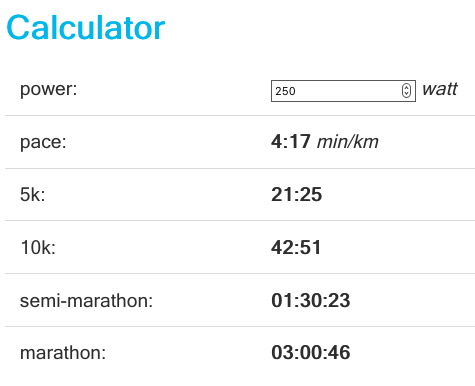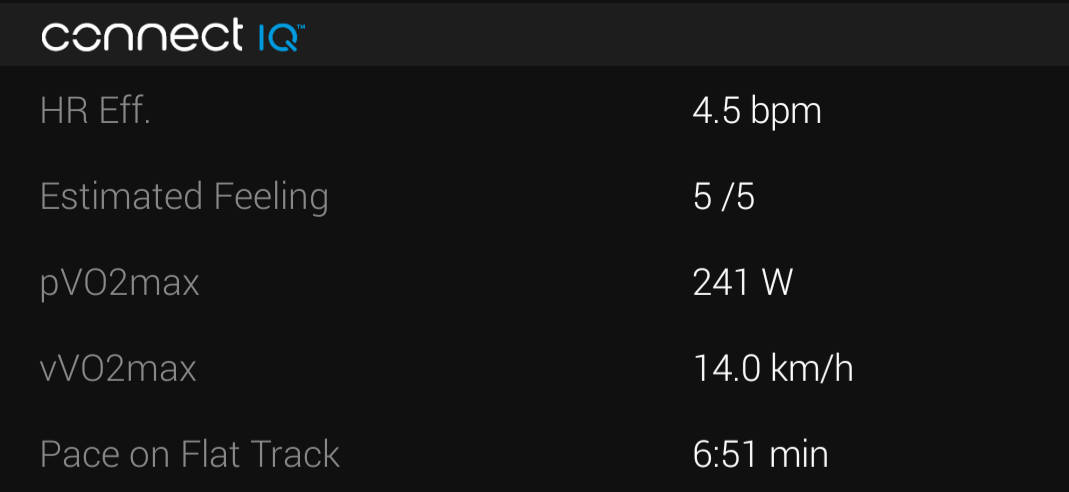# Estimation of the VVO2max Thanks to the Power and the Heart Rate

Jan 24, 2022

The vVO2max (velocity at maximal oxygen uptake) is the threshold speed beyond which oxygen consumption stop increasing. It is certainly possible to run faster but the energy consumed is no longer provided by aerobic process only but by the anaerobic process which generate lactic acid.

It is therefore also known as the speed of running at maximum oxygen consumption. The acronym vVO2max describes it well: vVO2max is the “velocity at VO2max”, the speed at VO2max.

vVO2max is interesting for three main reasons:

1. It allows you to define the intensity of the training stages based on on the pace. Classically, interval trainings are done between 100% and 105% of the vVO2max while endurance sessions are done between 65% and 70%.
2. It allows a (very) rough estimation of your running time:
Running Pace
Marathon 80% vVO2max
Half marathon 85% vVO2max
10 km 90% vVO2max
3. This is the maximum speed that can be maintained for 4 to 8 minutes. This information is particularly useful in uphill running as soon as you are able to convert convert this speed into the corresponding power value. Imagine, for example, a uphill of 3km, you know that if you exceed this power, you may not be able to maintain the actual speed.
Not uninteresting to manage the effort!

Moreover, we can also define the pVO2max, maximum aerobic power, which is nothing more than the power corresponding to the vVO2max on an athletic track.

There are a number of tests to determine your vVO2max. They are simple in theory, but as soon as you have to do them, it is less so.

Let’s take the case of the half-Cooper test. All you have to do is run for 6 minutes at full speed after a warm-up. Trivial you might think, but Guillaume Adam from RunMotion Coach reminded me of a rather important point:

The difficulty for the half-cooper test is to maintain a constant effort at high intensity, which athletes can do on the track.
But, it’s more for more experienced runners because running 6 minutes at full intensity is difficult for beginners.

For beginners, it is not easy and yet, it is precisely when you are a beginner that you need to have an idea of your level…

There are also various tests which increases the load step by step, like the Conconi test. But, I think that they substitute the difficulty of running hard for a few minutes by a complexity of the implementation.

Finally, a test is carried out at a precise moment and to be sure of the result, it must be repeated at least once after a few days of rest.

Hence the idea to completely free ourselves from these procedures and to use the data collected to deduce the vVO2max.

# Using existing algorithms

Before reinventing the wheel, one approach is to use the metrics calculated by the training platforms to deduce the vVO2max.

## Garmin’s VO2max

Garmin calculates a VO2max during training. There is a relation between vVO2max and VO2max known as the formula of Léger:

$$vVO2max = \frac{VO2max}{3.5} \quad km/h$$

Garmin evaluates my VO2max at 53, so I would have a vVO2max of 15.1km/h.

The problem with this formula is that the 3.5 factor is very approximative and is more or less applicable depending on the runner. For athletes, it is closer to 3.0. And in this case, my vVO2max would be 17.7km/h. The difference is huge and, moreover, the difference of 0.1 of this factor changes the vVO2max by 0.5km/h.

The inaccuracy of this formula is the first problem. The second is the estimation of VO2max. We simply do not know how the algorithm compute it. One might think that as long as it works, it is sufficient. But, how do we know if it gives a credible result without knowing its limitations and its assumptions?
But, even if this algorithm matches perfectly, Garmin only provides an integer integer as a result. This means that a VO2max of 53 is actually between 52.5 and 53.5.

In this case, if we do the calculation, by varying the factor of Léger between 3.4 and 3.6, and the VO2max between 52.5 and 53.5, we obtain a vVO2max between 14.6km/h and 15.7km/h. This is an error of 7% just by playing the last significant digit! And here we assume that the VO2max is evaluated with a good accuracy anyway. With other platforms, you can get a completely different VO2max. In my case, Run Motion Coach evaluates it at 49 with a vVO2max of 14km/h.

Conclusion: this method is simply not enough accurate.

## Stryd’s race prediction

Another approach is to use the platform prediction tool Stryd PowerCenter. The basic idea is to try to to have an estimate of a 1.5km run (the smallest possible distance for this tool) which generally corresponds to a run of 4 to 8 minutes. And the running speed for such period is assumed to be the vVO2max.

Stryd estimate also a power, which can also be considered to be the pVO2max, as well as a race duration. The vVO2max would then be:

$$vVO2max = \frac{5400}{minutes \times 60 + seconds} \qquad km/h$$ $$vVO2max = \frac{3355}{minutes \times 60 + seconds} \qquad mph$$

In my case, I get 235W and 06:39, which is a vVO2max of 13.5km/h. This value is much less fanciful than the Garmin one. And that can be explained very well.

First of all, Stryd’s race prediction is based on learning of the best times over a range of distances. So Stryd supposes that we run regularly at full speed over different short distances (interval trainings) or medium distances, (fartlek, for example). We cannot really qualify this algorithm with the term of predictor. It is rather an algorithm that searches in the last runs the best times for a distance equivalent to 1500m and indicates its duration. This is not quite truth because a mathematical model is used. But, the main idea is still valid.

In any case, it gives a good result if the critical power is correctly estimated. But, anyway, we must keep in mind that, because of its nature, the critical power is not really adapted to make predictions for short distance races. It corresponds rather to a power that can be maintained from 30 to 70 minutes. Predicting performance for a short effort with an indicator designed for the endurance is a bit questionable.

## The half-cooper test

To validate the principle, I performed a half-cooper test. It’s quite simple since I just have to warm up for 10 minutes and run as fast as possible for 6 minutes. The time of the 6 minutes gives directly the vVO2max by moving the decimal point.

I did 1.36km in 6 minutes, so the vVO2max is 13.6km/h. The average power, the pVO2max, was 231W. And we also get the maximum heart rate, in my case 174 beats/minute.

# Principle of the calculation of the vVO2max

The VO2max is the maximum consumption of oxygen by the aerobic pathway during of an effort. The vVO2max is the speed of the runner arrived at this maximum. The problem is that we can not make this measurement directly. It would have to analyze the gas exchange or make a blood analysis during effort. Unrealistic outside a laboratory!

One idea is to assume that if the runner is at his maximum VO2, he is also running at his maximum heart rate frequency. This is true for a large proportion of runners, especially beginners, but not for all! Indeed, what limits the VO2 is not necessarily the cardiovascular system, it can also be the ventilation, the muscular energy consumption or the amount of energy that the body can extract from fats or carbohydrates. For an athlete, the limiting factor is often not the cardiovascular system.

It is therefore necessary to check your session history to see if you have run at your maximum heart rate during a session (interval or short race of one or two kilometers).

Then it is just necessary to find the power you need to maintain for 4 minutes to reach this heart rate and then to deduce the speed. This algorithm is then nothing more than a simulation of a half-cooper test.

# A little math

Power Tool tries to estimate the heart rate using the formula:

$$HR = a_0 + \sum_{i=1}^{5}{ a_{3i-1} \times P[-15i\ldots 0] + a_{3i-2} \times \log( P[-15i\ldots 0] + 1 ) } + a_{3i} \times D[-15i\ldots 0]$$

P[a...b] is the average power from the relative time a to b. P[-15...0], P[-30...0] and P[-60...0], are the average power of the last 15, 30 and 60 seconds. D[a...b] is the standard deviation of the power over the interval [a...b].

We will assume that the power is kept as stable as possible, so D[a...b] is zero. Also P[a...b] is constant. So we simply note it P.

This gives the formula: $$HR = a_0 + ( \sum_{i=1}^{5}{ a_{3i-1} }) \times P + ( \sum_{i=1}^{5}{ a_{3i-2} }) \times \log( P + 1 )$$

It only remains to find the value of P for which HR is equal to the maximum heart rate. To do this, we can use the method of Newton. This gives us the pVO2max.
Then, to deduce the vVO2max, we simply calculate the equivalent pace on the track for the pVO2max.

# Implementation

## Principle

To determine your vVO2max by hand, simply

1. take your maximum heart rate,
2. deduce the pVO2max from the power/heart rate graph,
3. and finally, read the vVO2max from the power/pace curve.

Specifically, in my case, we obtain this analysis page.

We proceed as follows:

1. My maximum heart rate is 174 beats,
2. My pVO2max is obtained by finding the power associated with this frequency: 232W3. The vVO2max is the speed on the track corresponding to the vVO2max: 13.5 km/h## Implementation

Calculating by hand is fine, but you might as well let your watch do it…

The maximum heart rate is simply taken from the heart rate zones defined in the user profile. It is the high value of the zone 5.

Then, simply run for several outings at various speeds, best by following a training plan or do at least one interval session to be close to the maximum heart rate during at least 30s.

Power Tool calculates at the end of each run the vVO2max and the pVO2max and adds it to the statistics page of the session on Garmin Connect:Of course, the first values should be taken carefully as long as the prediction model doesn’t have learn enough information. But, after one or two weeks, an accurate value of the vVO2max should be computed.

# Conclusion

The prediction algorithm gave me a pVO2max of 232W and a vVO2max of 13.5km/h. This is very close to what is predicted by Stryd and measured by a Half-Cooper test: all 3 give the same value within 0.1 km/h! The margin of error is really negligible unlike a calculation based on the VO2max estimated by Garmin.

We can wonder what is the use of such a calculation if Stryd allows to determine the vVO2max by an indirect method using the run predictor.
The reason is that this method is based on the assumption that the power profile of the “Power Duratuion Curve” is significant. To archive this, you must have performed runs in the last two months at the maximum of your capabilities on the whole range of durations, from 30s up to one hour. If this is not the case the critical power is not correct.

The method used here is suitable for runners who prefer slow runs and it allows a quicker estimation of their vVO2max because only two to three split sessions are needed to get it.

Another interesting point is to check the validity of his critical power. Indeed, if two algorithms using two different methods give the same result, there is a good chance that it is correct. In my case, both algorithm give me an identical vVO2max. This means that the critical power is certainly estimated correctly.

Finally, we must not forget one thing, the vVO2max is an indicator for sessions of 5 minutes while the critical power is more around one hour. One is to be used for short runs, the other for endurance. Having an estimation of both is a good thing as indicator or to manage the effort for short or long sessions.